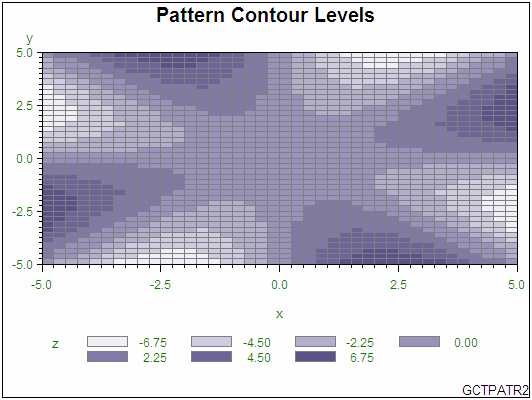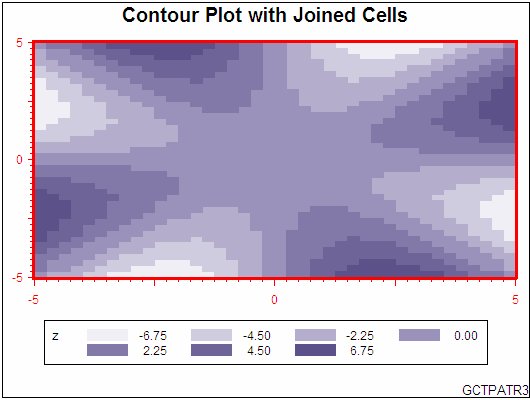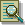The GCONTOUR Procedure

# Example 4: Using Patterns and Joins

 Procedure features: COUTLINE, CTEXT, HAXIS, JOIN, LEGEND, PATTERN Data Set SWIRL Sample library member: GCTPATJN

This example demonstrates the differences between using lines and patterns to represent contour levels. The first PLOT statement generates a plot with lines representing contour levels.

Line Contour LevelsThe second PLOT statement specifies the PATTERN option to fill and color contour levels. Additional PLOT statement options outline filled areas in gray and specify green text for all text on the axes and in the legend.

Pattern Contour LevelsThe third PLOT statement uses the JOIN option to combine adjacent grid cells with the same pattern to form a single pattern area. Additional options enhance the plot by modifying the axes and framing the legend.

Contour Plot with Joined Cells`goptions reset=all border;````data swirl; do x= -5 to 5 by 0.25; do y= -5 to 5 by 0.25; if x+y=0 then z=0; else z=(x*y)*((x*x-y*y)/(x*x+y*y)); output; end; end; run;``````title1 "Line Contour Levels"; footnote1 j=r "GCTPATR1";``````proc gcontour data=swirl; plot y*x=z; run; quit;``````title1 "Pattern Contour Levels"; footnote j=r "GCTPATR2";``````proc gcontour data=swirl; plot y*x=z / ctext=green coutline=gray pattern; run; quit;``````title "Contour Plot with Joined Cells"; footnote j=r "GCTPATR3";``````axis1 label=none value=("-5" '' "0" '' "5") color=red width=3; axis2 label=none value=("-5" '' "0" '' "5") color=red width=3; ````legend1 frame;````proc gcontour data=swirl; plot y*x=z / haxis=axis1 join legend=legend1 pattern vaxis=axis2; run; quit;```Previous Page | Next Page | Top of Page